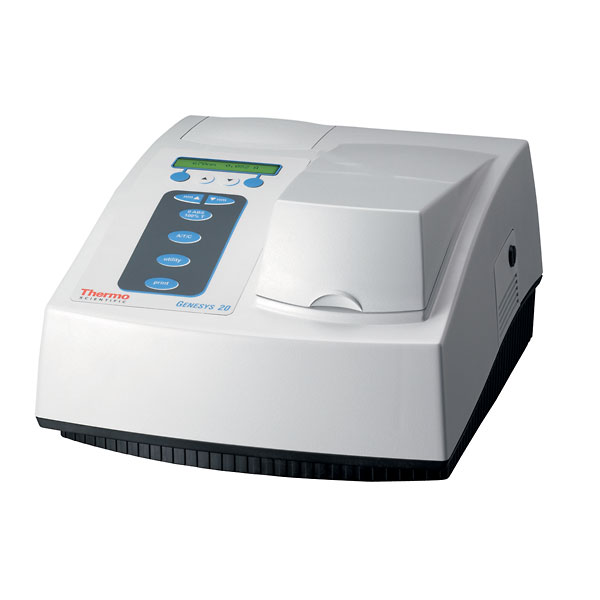ملتقى طلاب وطالبات جامعة الملك فيصل,جامعة الدمام[ كلية العلوم الطبية ] : Spectrophotometry
 التسجيل الكويزات إضافة كويز مواعيد التسجيل قائمة الأعضاء أعتبر مشاركات المنتدى مقروءة

 ملتقى طلاب وطالبات الكليات الصحية - جامعة الإمام عبدالرحمن ملتقى طلاب وطالبات الكليات الصحية التابعة لجامعة الإمام عبدالرحمن الدمام سابقاً

#1المشرفة العامة سابقاً ملتقى الفنون الأدبية بيانات الطالب: الكلية: IAU CAMS الدراسة: انتظام التخصص: SPECILIST OF CLS المستوى: خريج جامعي إحصائيات الموضوع: المشاهدات: 741 المشاركـات: 1 الملف الشخصي: رقم العضوية : 150409 تاريخ التسجيل: Thu Jul 2013 المشاركات: 21,933 الـجنــس : أنـثـى عدد الـنقـاط : 511920 مؤشر المستوى: 758SpectrophotometrySpectrophotometry Basic Principles Concepts A spectrophotometer is employed to measure the amount of light that a sample absorbs. The instrument operates by passing a beam of light through a sample and measuring the intensity of light reaching a detector. The beam of light consists of a stream of photons, represented by the purple balls in the simulation shown below. When a photon encounters an analyte molecule (the analyte is the molecule being studied), there is a chance the analyte will absorb the photon. This absorption reduces the number of photons in the beam of light, thereby reducing the intensity of the light beam. You can visualize this process by running the simulation shown below. Click on the Start button to start the simulation and the Stop button to stop the simulation. The light source is set to emit 10 photons per second. Watch the motion of the photons and observe how some of the photons are absorbed (removed) as the beam of light passes through the cell containing the sample solution. The intensity of the light reaching the detector is less than the intensity emitted by the light source. Experimental Procedure The following simulation illustrates the procedures for making spectrophotometric measurements. First, the intensity of light (I0) passing through a blank is measured. The intensity is the number of photons per second. The blank is a solution that is identical to the sample solution except that the blank does not contain the solute that absorbs light. This measurement is necessary, because the cell itself scatters some of the light. Second, the intensity of light (I) passing through the sample solution is measured. (In practice, instruments measure the power rather than the intensity of the light. The power is the energy per second, which is the product of the intensity (photons per second) and the energy per photon.) Third, the experimental data is used to calculate two quantities: the transmittance (T) and the absorbance (A). T = II0 A = - log10 T The transmittance is simply the fraction of light in the original beam that passes through the sample and reaches the detector. The remainder of the light, 1 - T, is the fraction of the light absorbed by the sample. (Do not confuse the transmittance with the temperature, which often is given the symbol T.) In most applications, one wishes to relate the amount of light absorbed to the concentration of the absorbing molecule. It turns out that the absorbance rather than the transmittance is most useful for this purpose. If no light is absorbed, the absorbance is zero (100% transmittance). Each unit in absorbance corresponds with an order of magnitude in the fraction of light transmitted. For A = 1, 10% of the light is transmitted (T = 0.10) and 90% is absorbed by the sample. For A = 2, 1% of the light is transmitted and 99% is absorbed. For A = 3, 0.1% of the light is transmitted and 99.9% is absorbed. Using the simulation below, perform the following steps: Measure the intensity of light passing through the blank. Measure the intensity of light passing through the sample. Calculate the transmittance. Calculate the absorbance. Note: For each measurement, run the simulation long enough to detect at least 1000 photons. There is substantial random error in the intensity, and the more photons that are counted, the lower the relative uncertainty in the results. RE: Virtual Chemistry Experiments2014- 11- 1 #2 صديقة الملتقى الترفيهي الملف الشخصي: رقم العضوية : 98465 تاريخ التسجيل: Sat Dec 2011 المشاركات: 17,628 الـجنــس : أنـثـى عدد الـنقـاط : 295031 مؤشر المستوى: 505بيانات الطالب: الكلية: جامعة الدراسة: انتساب التخصص: إدارة الأعمال المستوى: خريج جامعيرد: SpectrophotometryShare Share this post onDiggDel.icio.usTechnoratiTwitterالذين يشاهدون محتوى الموضوع الآن : 1 ( الأعضاء 0 والزوار 1)
 أدوات الموضوعمشاهدة صفحة طباعة الموضوعأرسل هذا الموضوع إلى صديقتعليمات المشاركة لا تستطيع إضافة مواضيع جديدة لا تستطيع الرد على المواضيع لا تستطيع إرفاق ملفات لا تستطيع تعديل مشاركاتك BB code is متاحة الابتسامات متاحة كود [IMG] متاحة كود HTML معطلةTrackbacks are متاحة Pingbacks are متاحة Refbacks are متاحة قوانين المنتدى

All times are GMT +3. الوقت الآن حسب توقيت السعودية: 03:56 PM.

 -- Arabic -- en أعلن معنا - اتصل بنا - الشكاوي والأقتراحات - ملتقى فيصل  - الأرشيف - إحصائيات الإعلانات - بيان الخصوصية - Privacy Policy - الأعلى